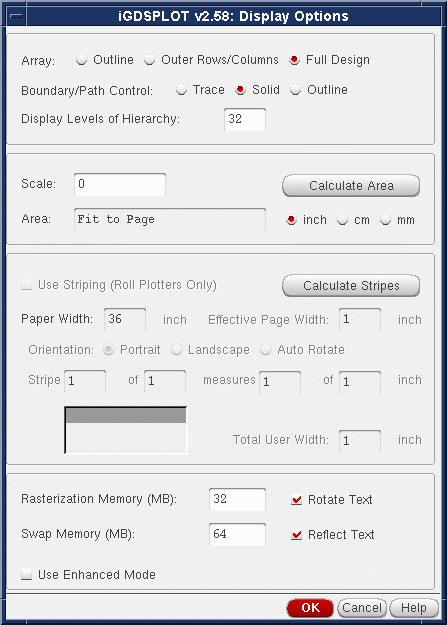## iGDSPLOT Display Options

 Clicking on the Display Options button from the main iGDPSLOT dialog opens the dialog box shown below:### Array

 Outline - Only a outline of the entire array is plotted. Outer Rows/Columns - Outer rows and colums are plotted. Full Design - All elements of an array are plotted.

### Boundary/Path Control

 Trace - Only the centerline of the path is plotted Solid - Paths are plotted as solid. Outline - Paths are plotted as if they were boundaries (default)

### Levels of Hierarchy

 0 - Everything is plotted from the current level all the way to the lowest level. [Default] n - Only n levels are plotted. For example if n=2 then only entities in the cell under consideration and entities in the cells directly referenced by this cell are plotted.

### Scale

 0 - Data extents are scaled to fit to the plottable area on the page. (Page size less the margins...) XXX - Data extents are scaled per user defined value. Note that no attempt is made to insure that the image will fit on the plottable area of the page.

### Calculate Area

Calculates the area needed to plot the chip based on the Window plotted and scale factor.

### Use Striping

This option should be activated when plotting a chip at high scale where the width of the paper
is not enough to fit the plot with one strip.
In this case the Auto Striping mode will get active and the interface will divide the plot to a number
of stripes based on the Roll paper width (which the user defines) and the Calculated Area.

### Paper Width

The width of the Roll on the plotter. Typical sizes are 24, 36, 42, 54, and 60 inches.

### Effective Page Width

Usually less than the Roll width because of margins (default is 1/2 inch margins on each side).

### Orientation

User can select between Portrait (0 rotation) or Landscape (90 degree rotation).
If the chip is not square, you should toggle between the two options to see which one produces less stripes.

### Stripe and Measure

Shows the user how many stripes are necessary to do the plot, and how big is each plot.

### Rasterization Memory

 XX - The number of MB allocated to holding the raster image of the page. Typically this value will range from 20-64 MB depending on the available RAM on the workstation. 64 MB can hold about a 36x36 image. If the image is larger than this then the program subdivides the image into smaller pieces and assembles the raster data on disk.